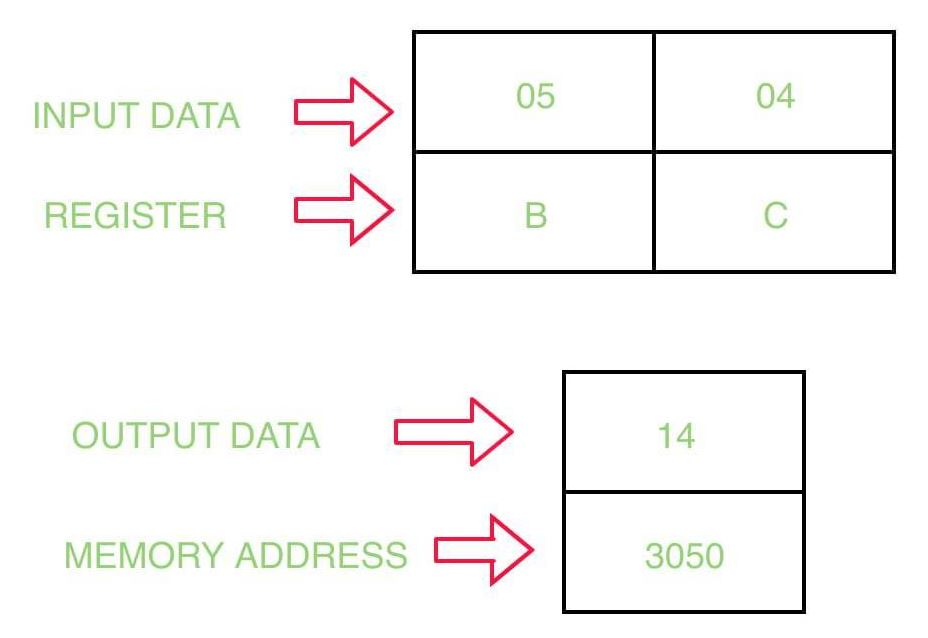# 8085 program to multiply two 8 bit numbers using logical instructions

Prerequisite – Logical instructions in 8085 microprocessor Problem – Write a assembly language program multiply two 8 bit numbers and store the result at memory address 3050 in 8085 microprocessor. Example –The value of accumulator(A) after using RLC instruction is:

`A = 2n*A`

Where n = number of times RLC instruction is used. Assumptions – Assume that the first number is stored at register B, and second number is stored at register C. And the result must not have any carry. Algorithm –

1. Assign the value 05 to register B
2. Assign the value 04 to register C
3. Move the content of B in A
4. Rotate accumulator left without carry
5. Rotate accumulator left without carry
6. Store the content of accumulator at memory address 3050
7. Halt of the program

Program –

Explanation –

1. MVI B 05: assign the value 05 to B register.
2. MVI C 04: assign the value 04 to C register.
3. MOV A, B: move the content of register B to register A.
4. RLC: rotate the content of accumulator left without carry.
5. RLC: rotate the content of accumulator left without carry.
6. STA 3050: store the content of register A at memory location 3050
7. HLT: stops the execution of the program.

• The program is simple and easy to understand since it only uses a few instructions.

• The program uses only logical instructions to perform the multiplication operation, which reduces the number of registers required.

• The program produces accurate results since it performs a series of bitwise operations to calculate the product.